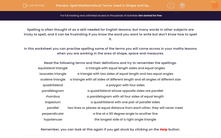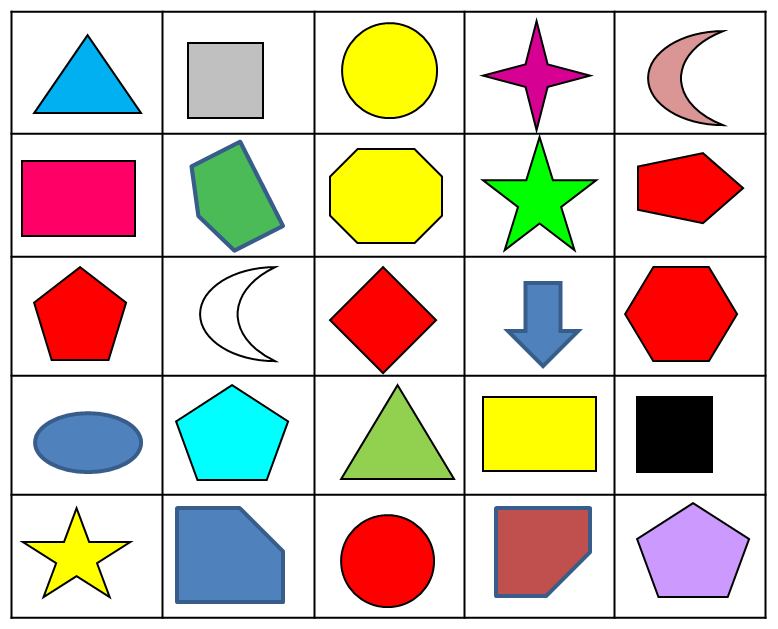# Spell Mathematical Terms Used with Shapes

In this worksheet, students will practise spelling terms related to shapes.Key stage:  KS 3

Curriculum topic:   Grammar and Vocabulary

Curriculum subtopic:   Vocabulary and Grammar Awareness

Difficulty level:#### Worksheet Overview

Spelling is often thought of as a skill needed for English lessons, but many words in other subjects are tricky to spell, and it can be frustrating if you know the word you want to write but don't know how to spell it.

In this activity, we will practise spelling some of the terms you will come across in your maths lessons when you are working in the area of shapes.Read the following terms and their definitions and try to remember the spellings.

 Equilateral triangle A triangle with equal length sides and equal angles Isosceles triangle A triangle with two sides of equal length and two equal angles Scalene triangle A triangle with all sides of different length and all angles of different size Quadrilateral A polygon with four sides Parallelogram A quadrilateral whose opposite sides are parallel Rhombus A parallelogram with all four sides of equal length Trapezium A quadrilateral with one pair of parallel sides Parallel Two lines or planes at equal distance from each other; they will never meet Perpendicular A line at a 90 degree angle to another line Hypotenuse The longest side of a right-angled triangle

Remember, you can look at this again if you get stuck by clicking on the red help button.